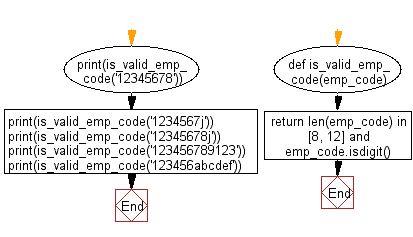﻿ Python: Check whether a given employee code is exactly 8 digits or 12 digits - w3resource# Python: Check whether a given employee code is exactly 8 digits or 12 digits

## Python Basic - 1: Exercise-87 with Solution

Write a Python program to check whether a given employee code is exactly 8 digits or 12 digits. Return True if the employee code is valid and False if it's not.

Sample Solution:

Python Code:

``````def is_valid_emp_code(emp_code):
return len(emp_code) in [8, 12] and emp_code.isdigit()
print(is_valid_emp_code('12345678'))
print(is_valid_emp_code('1234567j'))
print(is_valid_emp_code('12345678j'))
print(is_valid_emp_code('123456789123'))
print(is_valid_emp_code('123456abcdef'))
``````

Sample Output:

```True
False
False
True
False
```

Pictorial Presentation:Flowchart:Python Code Editor:

Have another way to solve this solution? Contribute your code (and comments) through Disqus.

What is the difficulty level of this exercise?

Test your Programming skills with w3resource's quiz.

﻿

## Python: Tips of the Day

What is the difference between Python's list methods append and extend?

append: Appends object at the end.

```x = [1, 2, 3]
x.append([4, 5])
print (x)
```

Output:

```[1, 2, 3, [4, 5]]
```

extend: Extends list by appending elements from the iterable.

```x = [1, 2, 3]
x.extend([4, 5])
print (x)
```

Output:

```[1, 2, 3, 4, 5]
```

Ref: https://bit.ly/2AZ6ZFq